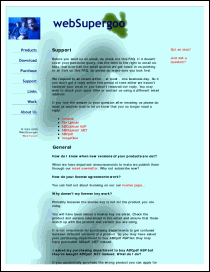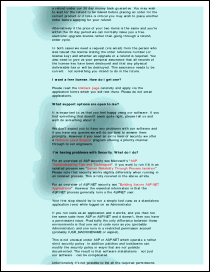LinkPages MethodConvert external links to internal links wherever possible.Syntax

 [C#] ```void LinkPages() void LinkPages(bool inPageNavigation)``` [Visual Basic] ```Sub LinkPages() Sub LinkPages(inPageNavigation as Boolean)```Params

 Name Description inPageNavigation If true then links will target particular locations on the destination page, if false then they will target just the page. Default false. return n/a.NotesExample

This example shows how to import an HTML page which uses named destinations.

We first create a Doc object and inset the edges a little so that the HTML will appear in the middle of the page. We assign the appropriate HTML options so that links will be rendered live.

[C#]
```Doc theDoc = new Doc(); theDoc.Rect.Inset(18, 18); theDoc.HtmlOptions.AddLinks = true;```
[Visual Basic]
```Dim theDoc As Doc = New Doc() theDoc.Rect.Inset(18, 18) theDoc.HtmlOptions.AddLinks = True```

We add the pages to the document.

[C#]
```int theID = theDoc.AddImageUrl("http://www.websupergoo.com/support.htm"); while (true) {   if (!theDoc.Chainable(theID))     break;   theDoc.Page = theDoc.AddPage();   theID = theDoc.AddImageToChain(theID); }```
[Visual Basic]
```Dim theID As Integer theID = theDoc.AddImageUrl("http://www.websupergoo.com/support.htm") While True   If Not theDoc.Chainable(theID) Then     Exit While   End If   theDoc.Page = theDoc.AddPage()   theID = theDoc.AddImageToChain(theID) End While```

The URL we've referenced makes extensive use of named destinations. We want these named destination links to take us between pages on the PDF rather than taking us to the original URL.

After adding the pages, we can flatten them. We can't do this until after the pages have been added because flattening will invalidate our previous ID and break the chain.

[C#]
```theDoc.HtmlOptions.LinkPages(); for (int i = 1; i <= theDoc.PageCount; i++) {   theDoc.PageNumber = i;   theDoc.Flatten(); }```
[Visual Basic]
```theDoc.HtmlOptions.LinkPages() For i As Integer = 1 To theDoc.PageCount   theDoc.PageNumber = i   theDoc.Flatten() Next```

Finally, we save.

[C#]```theDoc.Save(Server.MapPath("linkpages.pdf")); theDoc.Clear();```
[Visual Basic]
```theDoc.Save(Server.MapPath("linkpages.pdf")) theDoc.Clear()```

We get the following output. The links work – where possible – within the PDF.linkpages.pdf [Page 1]linkpages.pdf [Page 2]